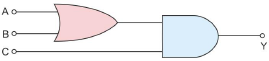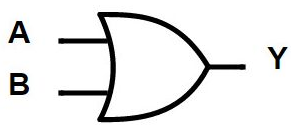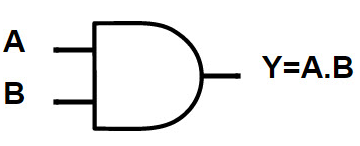# To get output 1 for the following circuit, the correct choice for the input is:Option 1) A = 0, B = 1, C = 0  Option 2) A = 1, B = 0, C = 0  Option 3) A = 1, B = 1, C = 0  Option 4) A = 0, B = 0, C = 1

OR gate -

Relation between input and output- wherein

A and B are input

Y is out put

AND Gate -- wherein

A and B are input

Y is out put

Output of the given circuit is

= (A+B)C

Option 1: A=0, B=1, C=0

= (0+1)0=0

Option 2: A=1, B=0, C=0

= (1+0)0=0

Option 3: A=1, B=1, C=0

= (1=1).0=0

Option 4: A=1, B=0, C=1

= (1+0)1=1

Option 1)

A = 0, B = 1, C = 0

This solution is incorrect.

Option 2)

A = 1, B = 0, C = 0

This solution is incorrect.

Option 3)

A = 1, B = 1, C = 0

This solution is incorrect.

Option 4)

A = 0, B = 0, C = 1

This solution is correct.

### Preparation Products

##### Rank Booster NEET 2021

This course will help student to be better prepared and study in the right direction for NEET..

₹ 13999/- ₹ 9999/-
##### Knockout NEET May 2021

An exhaustive E-learning program for the complete preparation of NEET..

₹ 22999/- ₹ 14999/-
##### Knockout NEET May 2022

An exhaustive E-learning program for the complete preparation of NEET..

₹ 34999/- ₹ 24999/-
##### Test Series NEET May 2021

Take chapter-wise, subject-wise and Complete syllabus mock tests and get in depth analysis of your test..

₹ 6999/- ₹ 4999/-